# Set Matrix Zeroes

Given an `m x n` integer matrix `matrix`, if an element is `0`, set its entire row and column to `0`'s, and return the matrix.

You must do it in place.

Example 1: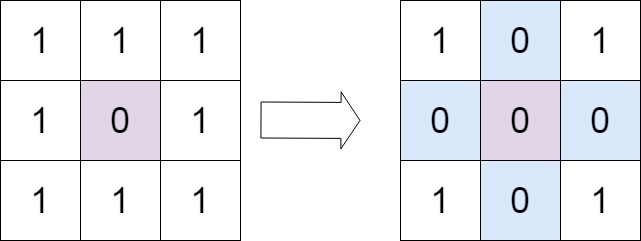```Input: matrix = [[1,1,1],[1,0,1],[1,1,1]]
Output: [[1,0,1],[0,0,0],[1,0,1]]
```

Example 2: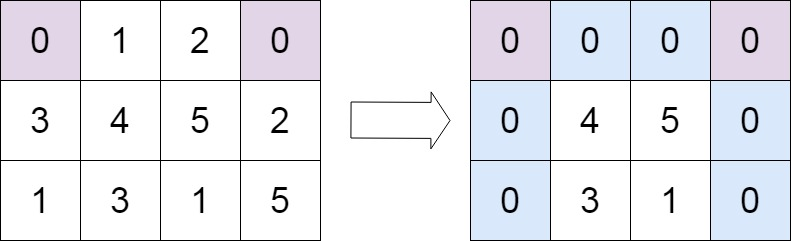```Input: matrix = [[0,1,2,0],[3,4,5,2],[1,3,1,5]]
Output: [[0,0,0,0],[0,4,5,0],[0,3,1,0]]
```

Constraints:

• `m == matrix.length`
• `n == matrix.length`
• `1 <= m, n <= 200`
• `-231 <= matrix[i][j] <= 231 - 1`

• A straightforward solution using `O(mn)` space is probably a bad idea.
• A simple improvement uses `O(m + n)` space, but still not the best solution.
• Could you devise a constant space solution?

### Solutions:

For to solve it in `O(mn)`, use a copy of given matrix and go through every element of original matrix and update the copy matrix's row and column to zero if a zero if found in original matrix.

### Space `O(m + n)` Solution:

```class Solution:
def setZeroes(self, matrix: List[List[int]]) -> None:
"""
Do not return anything, modify matrix in-place instead.
"""
ROWS, COLS = len(matrix), len(matrix)
rows =  * ROWS
cols =  * COLS
for r in range(ROWS):
for c in range(COLS):
if matrix[r][c] == 0:
rows[r] = 1
cols[c] = 1
for c in range(COLS):
for R in range(len(rows)):
if rows[R] == 1:
matrix[R][c] = 0
for r in range(ROWS):
for C in range(len(cols)):
if cols[C] == 1:
matrix[r][C] = 0
```

### Space `O(1)` Solution:

```class Solution:
def setZeroes(self, matrix: List[List[int]]) -> None:
"""
Do not return anything, modify matrix in-place instead.
"""
ROWS, COLS = len(matrix), len(matrix)

rowZero = False

for r in range(ROWS):
for c in range(COLS):
if matrix[r][c] == 0:
matrix[c] = 0
if r > 0:
matrix[r] = 0
else:
rowZero = True
for r in range(1, ROWS):
for c in range(1, COLS):
if matrix[c] == 0 or matrix[r] == 0:
matrix[r][c] = 0
if matrix == 0:
for r in range(ROWS):
matrix[r] = 0
if rowZero:
for c in range(COLS):
matrix[c] = 0

```Ashwini Verma on Jan 31, 2022 at 04:01 am

#### Post Comment

##### INTERVIEW EXPERIENCES##### Google 9+ Years experience Customer Solution Engineer##### UBS interview Questions ( PLSQL 6 Years Experience)##### LNT Infotech-Python Developer Interview Questions Experienced##### Mindfire Solutions Python developer Interview Questions Experienced##### Onit India Pvt Ltd - Python Developer Interview questions Experienced##### Incedo Interview questions Experienced Python Djnago##### Python Developer - Kapstone LLC Pune Location - Experienced Interview Questions##### NICE Interactive Solutions Interview Questions Experienced PL/SQL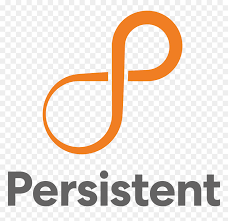##### Persistent Interview Experience C++##### Attra Interview Experience (PL/SQL 7 Years Experience)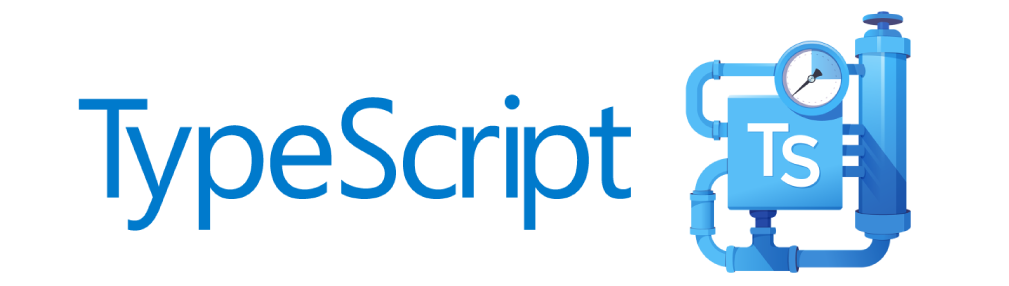##### TypeScript Programmning Language##### New HTML5 APIs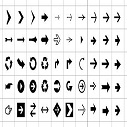##### HTML Arrow -> Range: Decimal 8592-8703. Hex 2190-21FF.##### How To Override One CSS Class With Another×